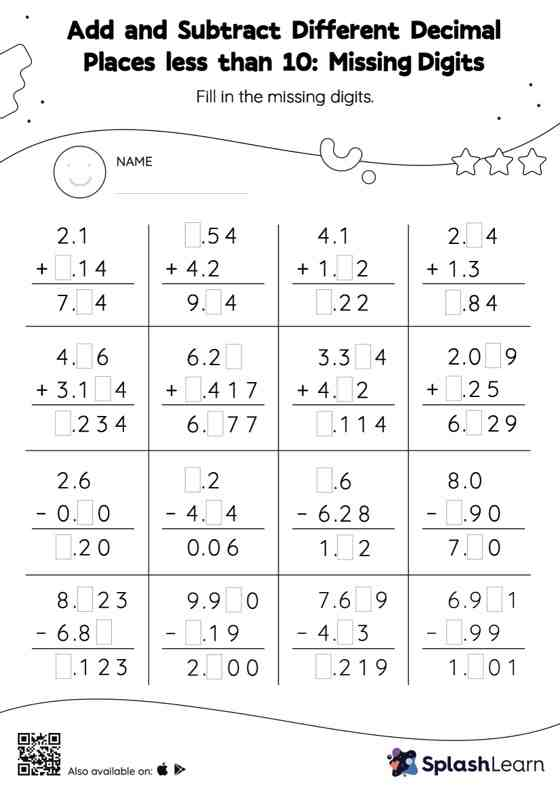# Add and Subtract Different Decimal Places less than 10: Missing Digits Worksheet

Home > Add and Subtract Different Decimal Places less than 10: Missing DigitsHelp your little one develop a knack for math with this add and subtract different decimal places less than 10 worksheet. Students align the decimal points and use zero as space holders to add and subtract decimals. Then they use the relationship between addition and subtraction to find the missing number in add and subtract different decimal places less than 10 worksheet. As the worksheet uses the column method, it is helpful in getting students toward higher accuracy, especially with bigger numbers and in scenarios where regrouping is required.